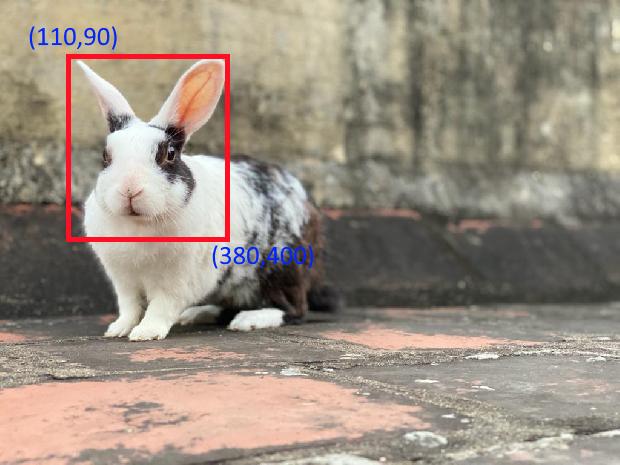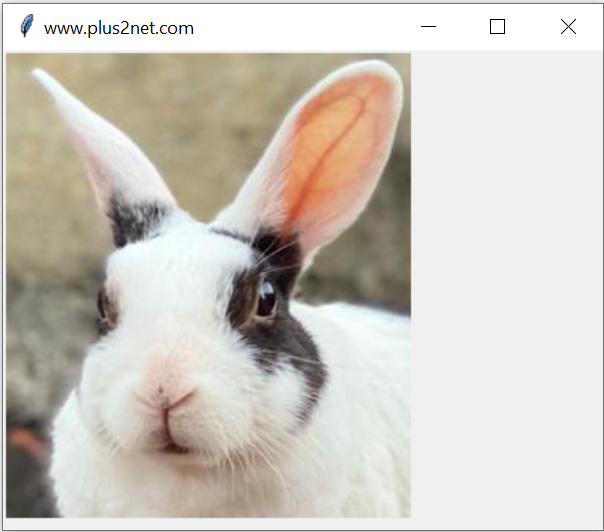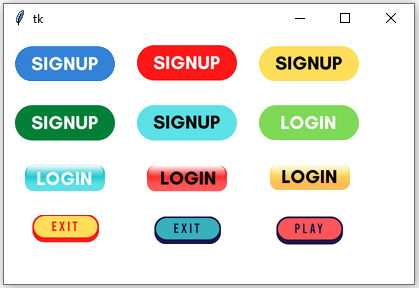# Python tkinter image resize

Basics of Python Tkinter Displaying Image• ### Video Tutorial on Tkinter Image resize

Original size of the image is 1024 x 768, we are reducing the size and displaying the image using one Label.
``````import tkinter as tk
from PIL import Image, ImageTk
my_w=tk.Tk()
my_w.geometry('400x300')
my_w.title('www.plus2net.com')
img_old=Image.open('D:\\images\\rabbit.jpg')
img_resized=img_old.resize((341,256)) # new width & height
my_img=ImageTk.PhotoImage(img_resized)

l1=tk.Label(my_w,image=my_img)
l1.grid(row=1,column=2)

my_w.mainloop()``````

## resize() with box option

We can use part of the picture or part of the area of the image with coordinates to resize. Here we will display the face only as resized output. Note the coordinates used.``````import tkinter as tk
from PIL import Image, ImageTk
my_w=tk.Tk()
my_w.geometry('400x320')
my_w.title('www.plus2net.com')
img_old=Image.open('D:\\images\\rabbit.jpg')

img_resized=img_old.resize((270,310),box=(110,90,380,400))
#im.save('D:\\images\\rabbit_face.jpg')
#im.show()
my_img=ImageTk.PhotoImage(img_resized)
l1=tk.Label(my_w,image=my_img)
l1.grid(row=1,column=2)
my_w.mainloop()``````## Maintaining Aspect ratio while resizing

Some time we need to maintain the same aspect ratio while resizing the image. In such cases we have to read the height and width of the image and then change the values equally. By using int() we are converting the float output to integer.
``````import tkinter as tk
from PIL import Image, ImageTk
my_w=tk.Tk()
my_w.geometry('400x300')
my_w.title('www.plus2net.com')
img_old=Image.open('D:\\images\\rabbit.jpg')
width, height = img_old.size
width_new=int(width/3)
height_new=int(height/3)
img_resized=img_old.resize((width_new,height_new))
my_img=ImageTk.PhotoImage(img_resized)
l1=tk.Label(my_w,image=my_img)
l1.grid(row=1,column=2)
my_w.mainloop()``````

## Colourful Buttons by using imagesSubscribe to our YouTube Channel here

## Subscribe

* indicates required
Subscribe to plus2netplus2net.com